## 2008年9月22日星期一

### SSAO 新進展

Yeah! 利用了法線緩衝所提供的資訊後， SSAO (屏幕空間環境光遮蔽) 的效果迫真了許多。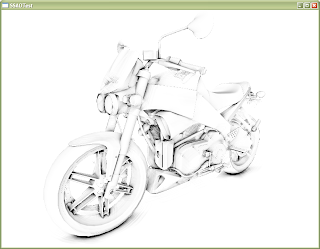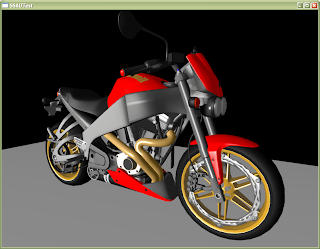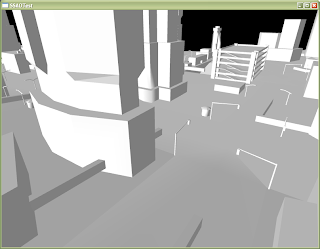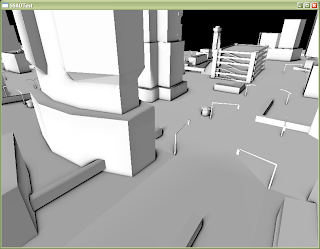1. 兩像素於三圍空間中的位置；深度較淺的像素會遮蔽較深的像素，而遮蔽的程度就取決於距離。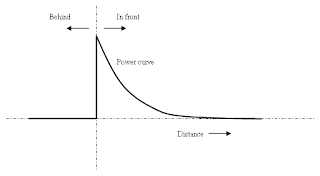2. 兩像素的法線內積 (Dot product)；面向面的像素會比面向同一方向的像素較接觸不到外來的光線。`uniform sampler2DRect texColor; // Color textureuniform sampler2DRect texDepth; // Depth textureuniform sampler2DRect texNormal;// Normal textureuniform vec2 camerarange = vec2(1.0, 500);varying vec2 texCoord;const float aoCap = 1.0;float aoMultiplier = 1000.0;float pw = 1.0;  // Use (1.0 / screensize.x) for GL_TEXTURE2Dfloat ph = 1.0;float readDepth(in vec2 coord){ float nearZ = camerarange.x; float farZ = camerarange.y; float posZ = texture2DRect(texDepth, coord).x; return (2.0 * nearZ) / (nearZ + farZ - posZ * (farZ - nearZ));}vec3 readNormal(in vec2 coord){ return normalize(2 * (texture2DRect(texNormal, coord).xyz - 1));}float compareDepths(in float depth1, in float depth2){ float depthDiff = depth1 - depth2; const float aorange = 10.0;  // Units in space the AO effect extends to (this gets divided by the camera far range) float diff = clamp(1.0 - depthDiff * (camerarange.y - camerarange.x) / aorange, 0.0, 1.0); return min(aoCap, max(0.0, depthDiff) * aoMultiplier) * diff;}float calAO(float depth, vec3 normal, float dw, float dh){ vec2 coord = vec2(texCoord.x + dw, texCoord.y + dh); float angleFactor = 1 - dot(normal, readNormal(coord)); if(length(normal) == 0) angleFactor = 0; return angleFactor * compareDepths(depth, readDepth(coord));}void main(void){ float depth = readDepth(texCoord); float ao = 0.0; vec3 normal = readNormal(texCoord); for(int i=0; i<8; ++i) {  ao += calAO(depth, normal, pw, ph);  ao += calAO(depth, normal, pw, -ph);  ao += calAO(depth, normal, -pw, ph);  ao += calAO(depth, normal, -pw, -ph);  pw *= 1.4;  ph *= 1.4;  aoMultiplier /= 1.5; } ao *= 2.0; gl_FragColor = vec4(1.0 - ao) * texture2DRect(texColor, texCoord);}`

#### 8 則留言:

1.推一個~ 加油

2.為甚麼「可惜再沒有人和我分享這份喜悅」呢? 我都為你感到圖形編程的樂趣而開心。

有幾點想討論一下，雖然我還未做過SSAO:
1. 實作中用了 linear 的 depth difference，而圖表中是 curve? 有沒有想過怎樣的數式才比較 physically correct? 是否應該是1/(4 pi r^2)

2. angleFactor 現時是 1-(N1.N2). 我感覺這樣好像不對, 例如正方體的兩個相隣的面，1-(N1.N2)= 1，但這兩個面是不應該產生AO效果。印像中 Crysis 的文章中是利用 surface normal 來比較附近的 position samples 是否在該 surface 的 half-space 裡。這會比較接近 AO 旳意義。

3. clamp(x, 0, 1) = saturate(x). Cg 裡建議用最簡單的函式，因為可能會有相應的 instruction，執行速度比較高。

4. 如果要比較不同SSAO的計算方法的好壞，最好是渲染一個用 AO (非 SSAO)的圖片作比較。嗯...這裡越來越像做 research...

我都想快些在我的引擊中做 Graphics...

3.嗯，Milo 所言甚是。
我只去思索大概的趨勢，還沒有深究當中的物理真實性。
暫時效果加性能可以就了事，騙不過 Milo 的眼睛但騙得過別人的就可以 :)
全因太過急切想完成一些 effect，日後有時間才深究深究。

4.阿...查考過 GLSL 是沒有 saturate() 的。

5.感覺很好
電單車的detail都出來了

還是對SSAO的中文名稱有些不習慣...

下一次會做甚麼呢?
其他 post rendering?

6.你好，

我是從 Percy 的部落格連結過來的。這篇 SSAO 的實作效果很不賴哪，不知道實際執行起來的效能如何呢？

另外，那篇 StarCraft2 的 paper 真的非常有趣。很久沒關注 SIGGRAPH，沒想到 Blizzard 會釋出有關遊戲圖像的論文，非得仔細讀讀才行。

最後想請問，不知道能不能將你的部落格加入蔽站的「連結」以及「聯播」頁面中？

謝謝 ^^

7.半路你好呀，很久以前已從 Milo 得知你的部落格哩! 無論翻譯或者自創的都那麼有水準，佩服佩服!

至於那個不甚正規的 SSAO，它在我的 9600GT 上運行 800x600 時有 140 FPS，並且相信 texture fetch 是瓶頸。

求之不得啦!

8.你好啊，
最近才打算嘗試看看SSAO的效果，沒想到在Blog晃著晃著，就在這裡看到好東西了。

希望以後能有機會多交流交流喔